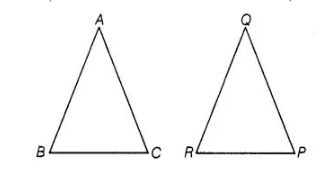# If in two

Question:

If in two $\triangle \mathrm{PQR}, \frac{A B}{Q R}=\frac{B C}{P R}=\frac{C A}{P Q}$,then

(a)Δ PQR~Δ CAB

(b) Δ PQR ~ Δ ABC

(c)Δ CBA ~ Δ PQR

(d) Δ BCA ~ Δ PQR

Solution:

(a) Given, in two $\triangle \mathrm{ABC}$ and $\triangle \mathrm{PQR}, \frac{A B}{Q R}=\frac{B C}{P R}=\frac{C A}{P Q}$

which shows that sides of one triangle are proportional to the side of the other triangle, then their corresponding angles are also equal, so by SSS

similarity, triangles are similar.

i.e.,                                           Δ CAB ∼ Δ PQR